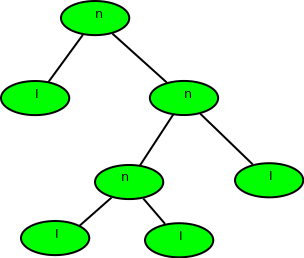# Calculate depth of a full Binary tree from Preorder

• Difficulty Level : Medium
• Last Updated : 06 Jul, 2022

Given preorder of a binary tree, calculate its depth(or height) [starting from depth 0]. The preorder is given as a string with two possible characters.

1. ‘l’ denotes the leaf
2. ‘n’ denotes internal node

The given tree can be seen as a full binary tree where every node has 0 or two children. The two children of a node can ‘n’ or ‘l’ or mix of both.

Examples :

```Input  : nlnll
Output : 2
Explanation :``````Input  : nlnnlll
Output : 3```Preorder of the binary tree is given so traverse

Also, we would be given a string of char (formed of ‘n’ and ‘l’), so there is no need to implement tree also.

The recursion function would be:

1. Base Case: return 0; when tree[i] = ‘l’ or i >= strlen(tree)
2. find_depth( tree[i++] ) //left subtree
3. find_depth( tree[i++] ) //right subtree

Where i is the index of the string tree.

Implementation:

## C++

 `// C++ program to find height of full binary tree` `// using preorder` `#include ` `using` `namespace` `std;`   `// function to return max of left subtree height` `// or right subtree height` `int` `findDepthRec(``char` `tree[], ``int` `n, ``int``& index)` `{` `    ``if` `(index >= n || tree[index] == ``'l'``)` `        ``return` `0;`   `    ``// calc height of left subtree (In preorder` `    ``// left subtree is processed before right)` `    ``index++;` `    ``int` `left = findDepthRec(tree, n, index);`   `    ``// calc height of right subtree` `    ``index++;` `    ``int` `right = findDepthRec(tree, n, index);`   `    ``return` `max(left, right) + 1;` `}`   `// Wrapper over findDepthRec()` `int` `findDepth(``char` `tree[], ``int` `n)` `{` `    ``int` `index = 0;` `    ``return` `findDepthRec(tree, n, index);` `}`   `// Driver program` `int` `main()` `{` `    ``// Your C++ Code` `    ``char` `tree[] = ``"nlnnlll"``;` `    ``int` `n = ``strlen``(tree);`   `    ``cout << findDepth(tree, n) << endl;`   `    ``return` `0;` `}`

## Java

 `// Java program to find height ` `// of full binary tree using` `// preorder` `import` `java .io.*;`   `class` `GFG ` `{` `    ``// function to return max ` `    ``// of left subtree height` `    ``// or right subtree height` `    ``static` `int` `findDepthRec(String tree, ` `                            ``int` `n, ``int` `index)` `    ``{` `        ``if` `(index >= n || ` `            ``tree.charAt(index) == ``'l'``)` `            ``return` `0``;`   `        ``// calc height of left subtree ` `        ``// (In preorder left subtree ` `        ``// is processed before right)` `        ``index++;` `        ``int` `left = findDepthRec(tree, ` `                                ``n, index);`   `        ``// calc height of` `        ``// right subtree` `        ``index++;` `        ``int` `right = findDepthRec(tree, n, index);`   `        ``return` `Math.max(left, right) + ``1``;` `    ``}`   `    ``// Wrapper over findDepthRec()` `    ``static` `int` `findDepth(String tree,` `                         ``int` `n)` `    ``{` `        ``int` `index = ``0``;` `        ``return` `(findDepthRec(tree, ` `                             ``n, index));` `    ``}`   `    ``// Driver Code` `    ``static` `public` `void` `main(String[] args)` `    ``{` `        ``String tree = ``"nlnnlll"``;` `        ``int` `n = tree.length();` `        ``System.out.println(findDepth(tree, n));` `    ``}` `}`   `// This code is contributed` `// by anuj_67.`

## Python3

 `#Python program to find height of full binary tree ` `# using preorder` `    `  `# function to return max of left subtree height ` `# or right subtree height ` `def` `findDepthRec(tree, n, index) :`   `    ``if` `(index[``0``] >``=` `n ``or` `tree[index[``0``]] ``=``=` `'l'``):` `        ``return` `0`   `    ``# calc height of left subtree (In preorder ` `    ``# left subtree is processed before right) ` `    ``index[``0``] ``+``=` `1` `    ``left ``=` `findDepthRec(tree, n, index) `   `    ``# calc height of right subtree ` `    ``index[``0``] ``+``=` `1` `    ``right ``=` `findDepthRec(tree, n, index)` `    ``return` `(``max``(left, right) ``+` `1``)`   `# Wrapper over findDepthRec() ` `def` `findDepth(tree, n) :`   `    ``index ``=` `[``0``] ` `    ``return` `findDepthRec(tree, n, index) `   `        `  `# Driver program to test above functions ` `if` `__name__ ``=``=` `'__main__'``:` `    ``tree``=` `"nlnnlll"` `    ``n ``=` `len``(tree) `   `    ``print``(findDepth(tree, n))`   `# This code is contributed by SHUBHAMSINGH10`

## C#

 `// C# program to find height of` `// full binary tree using preorder` `using` `System;`   `class` `GFG {`   `    ``// function to return max of left subtree` `    ``// height or right subtree height` `    ``static` `int` `findDepthRec(``char``[] tree, ``int` `n, ``int` `index)` `    ``{` `        ``if` `(index >= n || tree[index] == ``'l'``)` `            ``return` `0;`   `        ``// calc height of left subtree (In preorder` `        ``// left subtree is processed before right)` `        ``index++;` `        ``int` `left = findDepthRec(tree, n, index);`   `        ``// calc height of right subtree` `        ``index++;` `        ``int` `right = findDepthRec(tree, n, index);`   `        ``return` `Math.Max(left, right) + 1;` `    ``}`   `    ``// Wrapper over findDepthRec()` `    ``static` `int` `findDepth(``char``[] tree, ``int` `n)` `    ``{` `        ``int` `index = 0;` `        ``return` `(findDepthRec(tree, n, index));` `    ``}`   `    ``// Driver program` `    ``static` `public` `void` `Main()` `    ``{` `        ``char``[] tree = ``"nlnnlll"``.ToCharArray();` `        ``int` `n = tree.Length;` `        ``Console.WriteLine(findDepth(tree, n));` `    ``}` `}`   `// This code is contributed by vt_m.`

## Javascript

 ``

Output

`3`

Time Complexity: O(N)
Auxiliary Space: O(1)

This article is contributed by Shubham Gupta. If you like GeeksforGeeks and would like to contribute, you can also write an article using write.geeksforgeeks.org or mail your article to review-team@geeksforgeeks.org. See your article appearing on the GeeksforGeeks main page and help other Geeks.

My Personal Notes arrow_drop_up
Recommended Articles
Page :